# Weak convergence of probability measures

(diff) ← Older revision | Latest revision (diff) | Newer revision → (diff)
The general setting for weak convergence of probability measures is that of a complete separable metric space(cf. also Complete space; Separable space),being the metric, with probability measures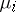,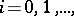defined on the Borel sets of. It is said that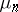converges weakly to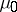inif for every bounded continuous functiononone has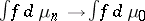as. If random elements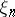,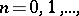taking values inare such that the distribution ofis,one writes, and says thatconverges in distribution to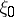ifconverges weakly to(cf. also Convergence in distribution).
The metric spaces in most common use in probability are,-dimensional Euclidean space,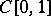, the space of continuous functions on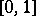, and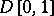, the space of functions onwhich are right continuous with left-hand limits.
Weak convergence in a suitably rich metric space is of considerably greater use than that in Euclidean space. This is because a wide variety of results on convergence in distribution oncan be derived from it with the aid of the continuous mapping theorem, which states that if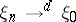in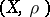and the mapping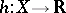is continuous (or at least is measurable and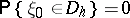, where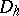is the set of discontinuities of), then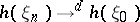. In many applications the limit random element is Brownian motion, which has continuous paths with probability one.
One of the most fundamental weak convergence results is Donsker's theorem for sums,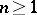, of independent and identically-distributed random variableswith,. This can be framed inby setting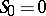and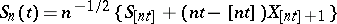,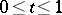, wheredenotes the integer part of. Then Donsker's theorem asserts that, where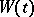is standard Brownian motion. Application of the continuous mapping theorem then readily provides convergence-in-distribution results for functionals such as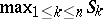,,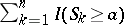, and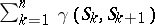, whereis the indicator function and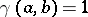ifandotherwise.